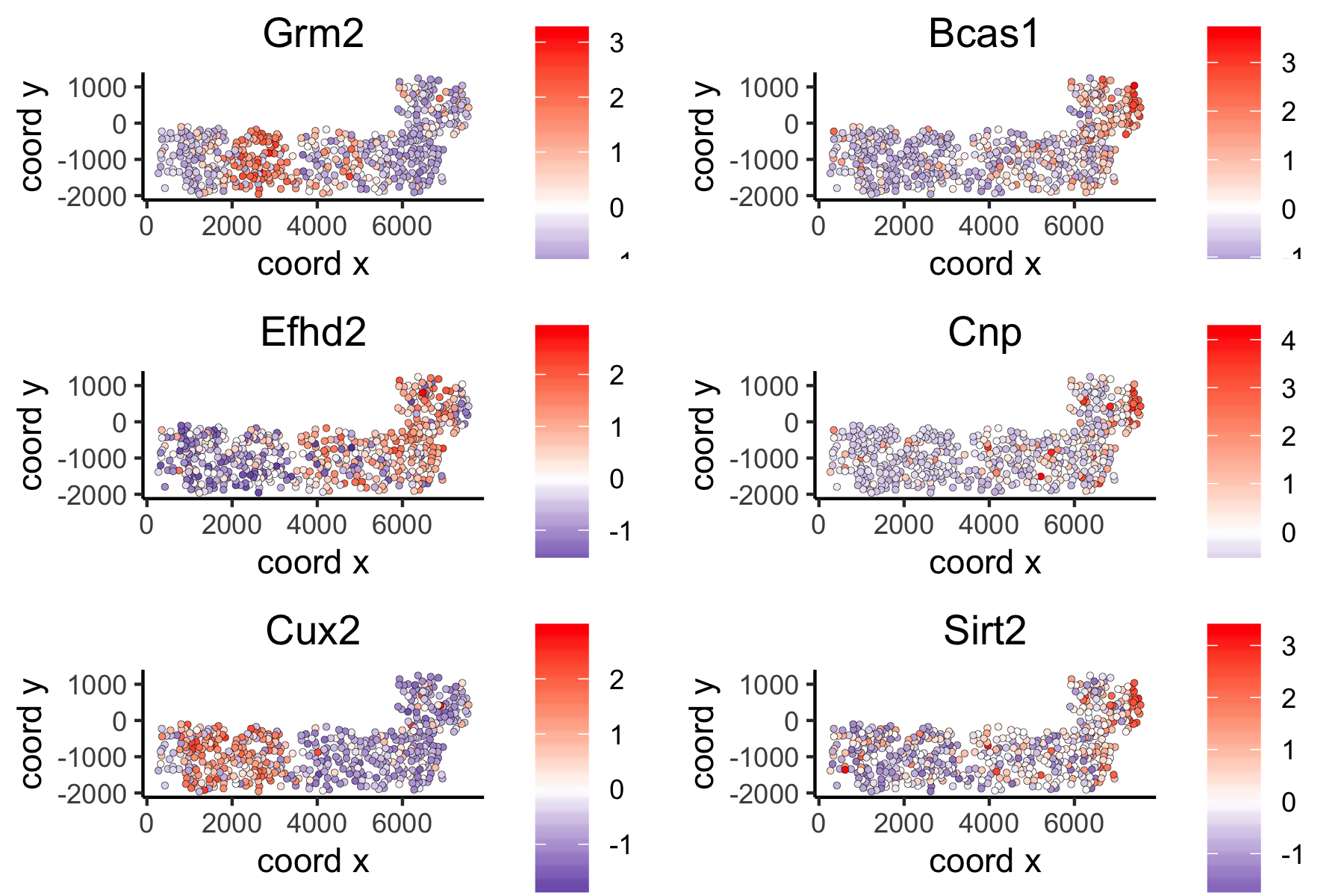# How to Test and Store Multiple Parameters or Analyses?¶

The default Giotto workflow is similar to other scRNA-seq workflows and does not require you to provide a custom name for each analysis (e.g. PCA, UMAP, …), but running an analysis twice will overwrite the previous results with a warning.

However, there are situations where being able to run and store multiple analyses can be advantageous:

• Test multiple parameters for a single analysis

• Test multiple combinations across functions (See Example: hvg->pca->umap)

• Use different output results as input for downstream analyses (See Example: spatial genes)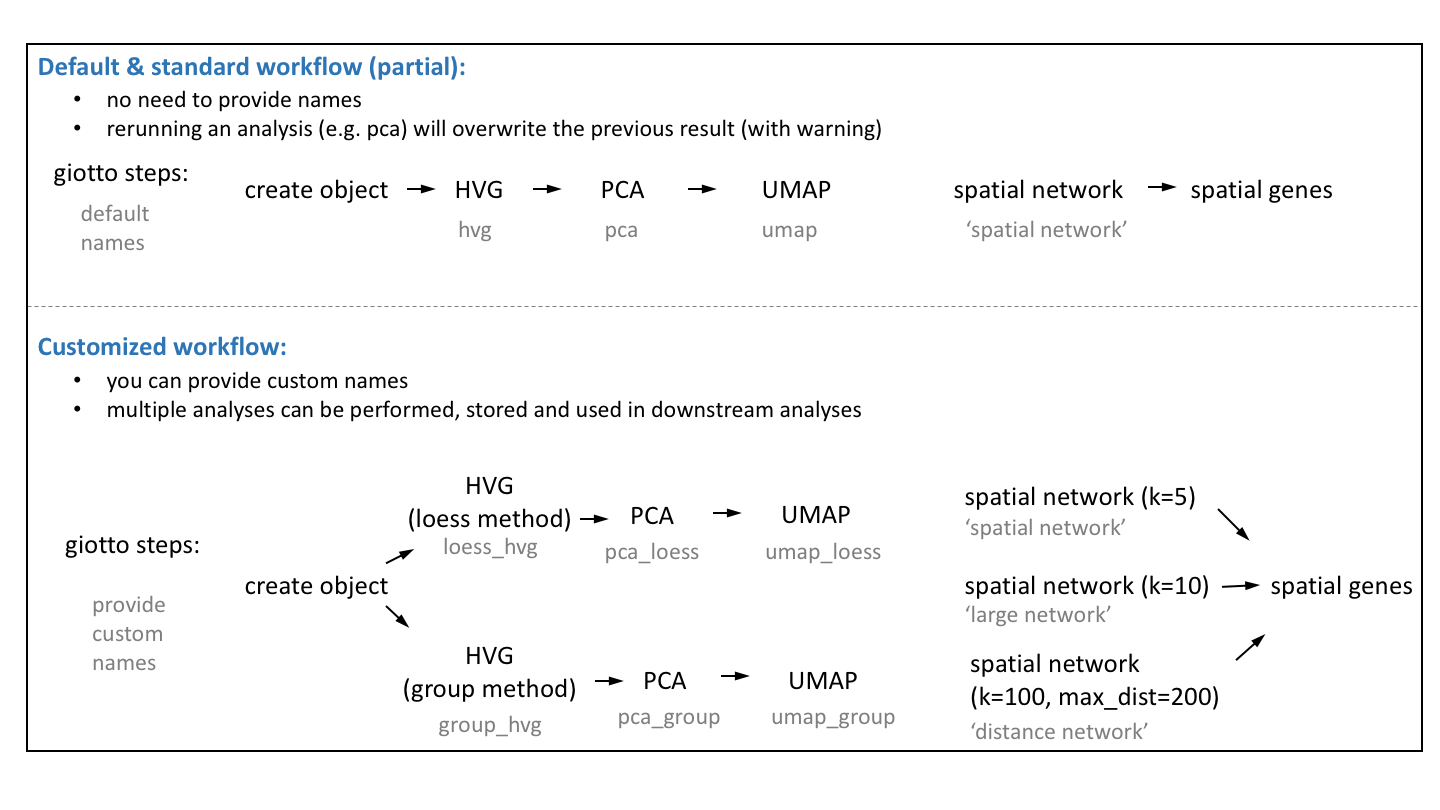We will use the seqFish+ somatosensory cortex as an example dataset after creating and processing a Giotto object.

## 1. Calculate Highly Variable Genes (2 Methods)¶

```# using the loess method
VC_test <- calculateHVG(gobject = VC_test,
method = 'cov_loess', difference_in_cov = 0.1,
HVGname = 'loess_hvg')
```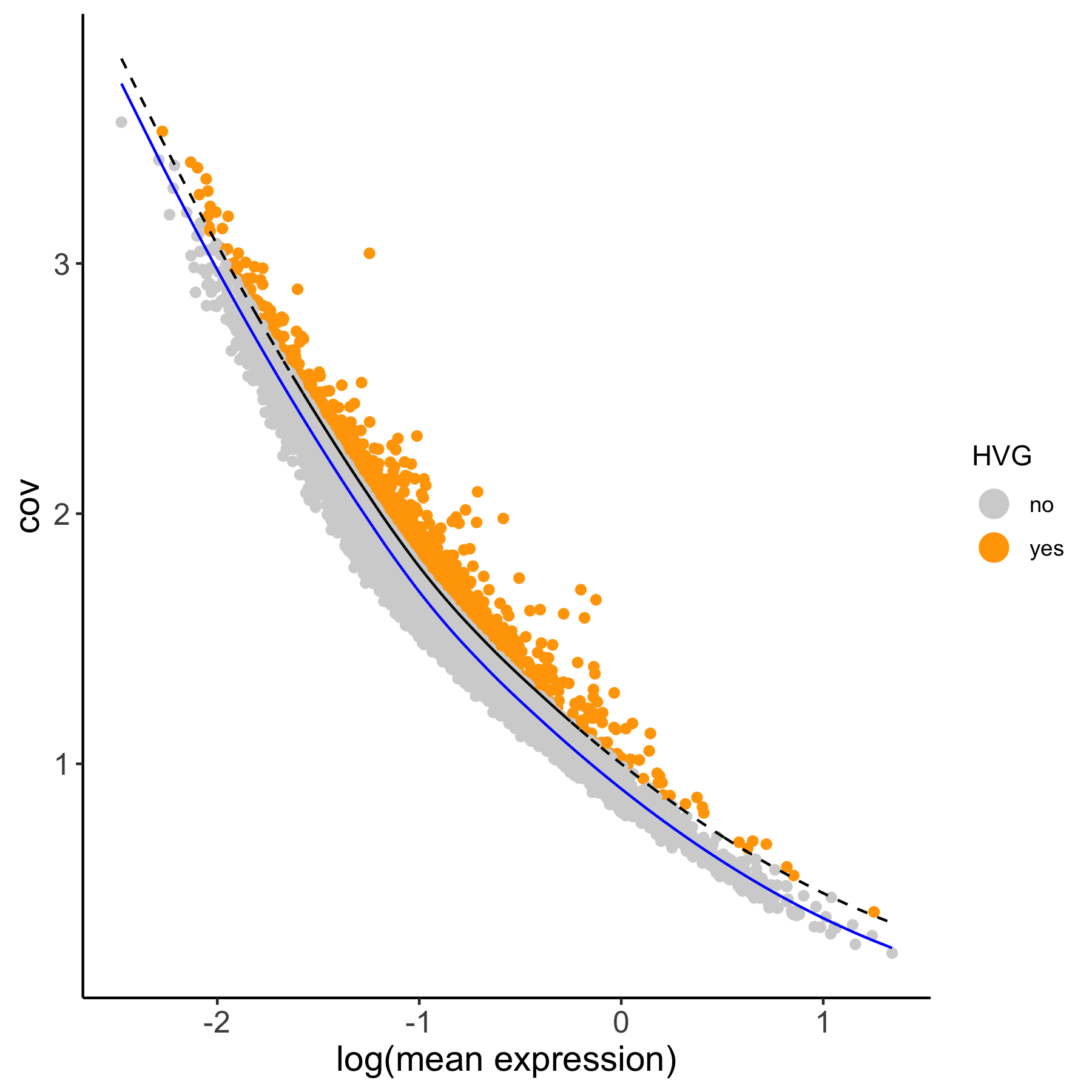```# using the expression groups method
VC_test <- calculateHVG(gobject = VC_test
, method = 'cov_group', zscore_threshold = 1,
HVGname = 'group_hvg')
```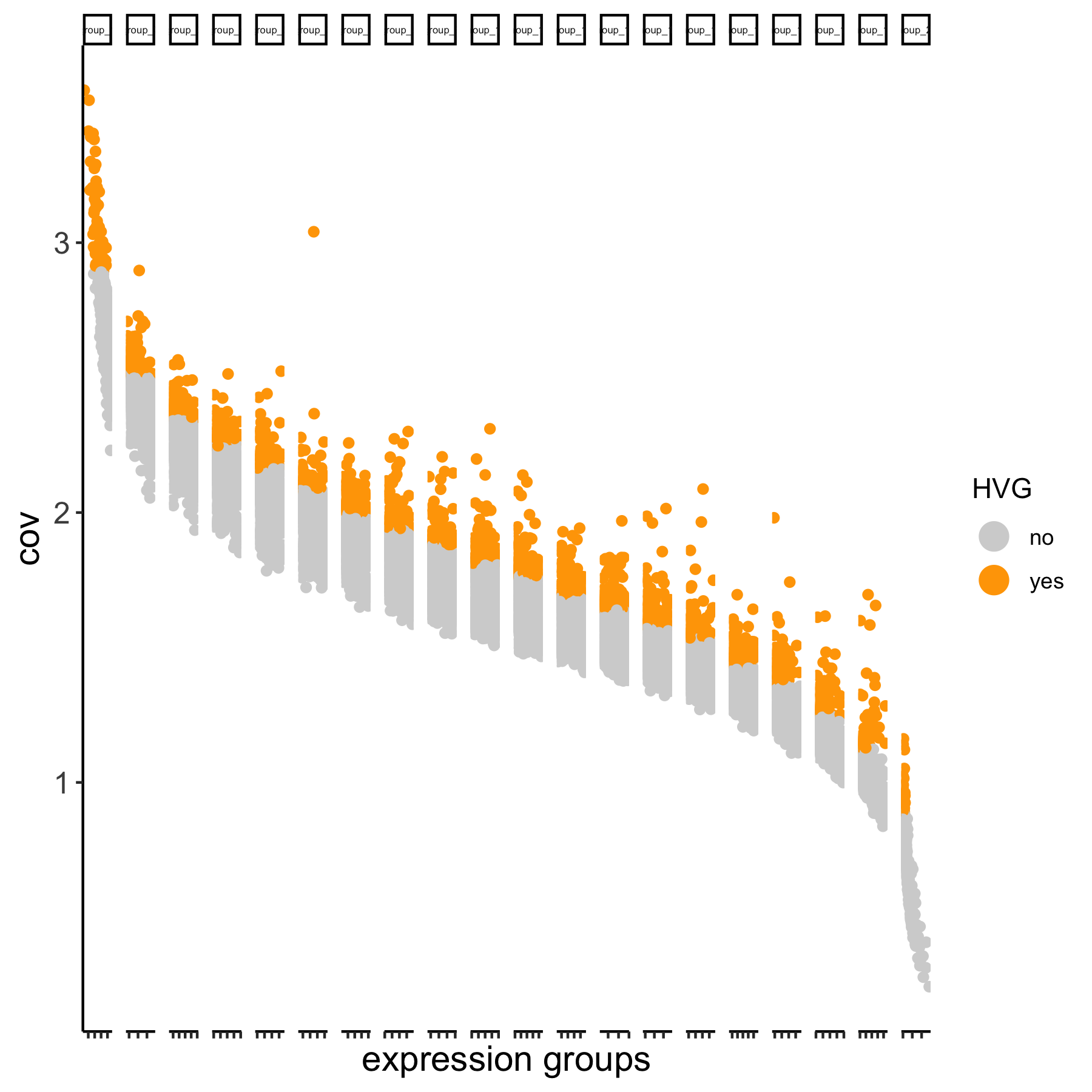```# compare the highly variable genes between two methods
```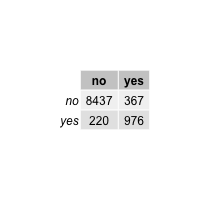## 2. Perform Multiple PCAs¶

• Using the 2 different HVG sets (loess_genes and group_genes)

• Store PCA results using custom names (‘pca_loess’ and ‘pca_group’)

• Plot PCA results

```## 2. PCA ##
# pca with genes from loess
VC_test <- runPCA(gobject = VC_test, genes_to_use = loess_genes, name = 'pca_loess', scale_unit = F)
plotPCA(gobject = VC_test, dim_reduction_name = 'pca_loess')
```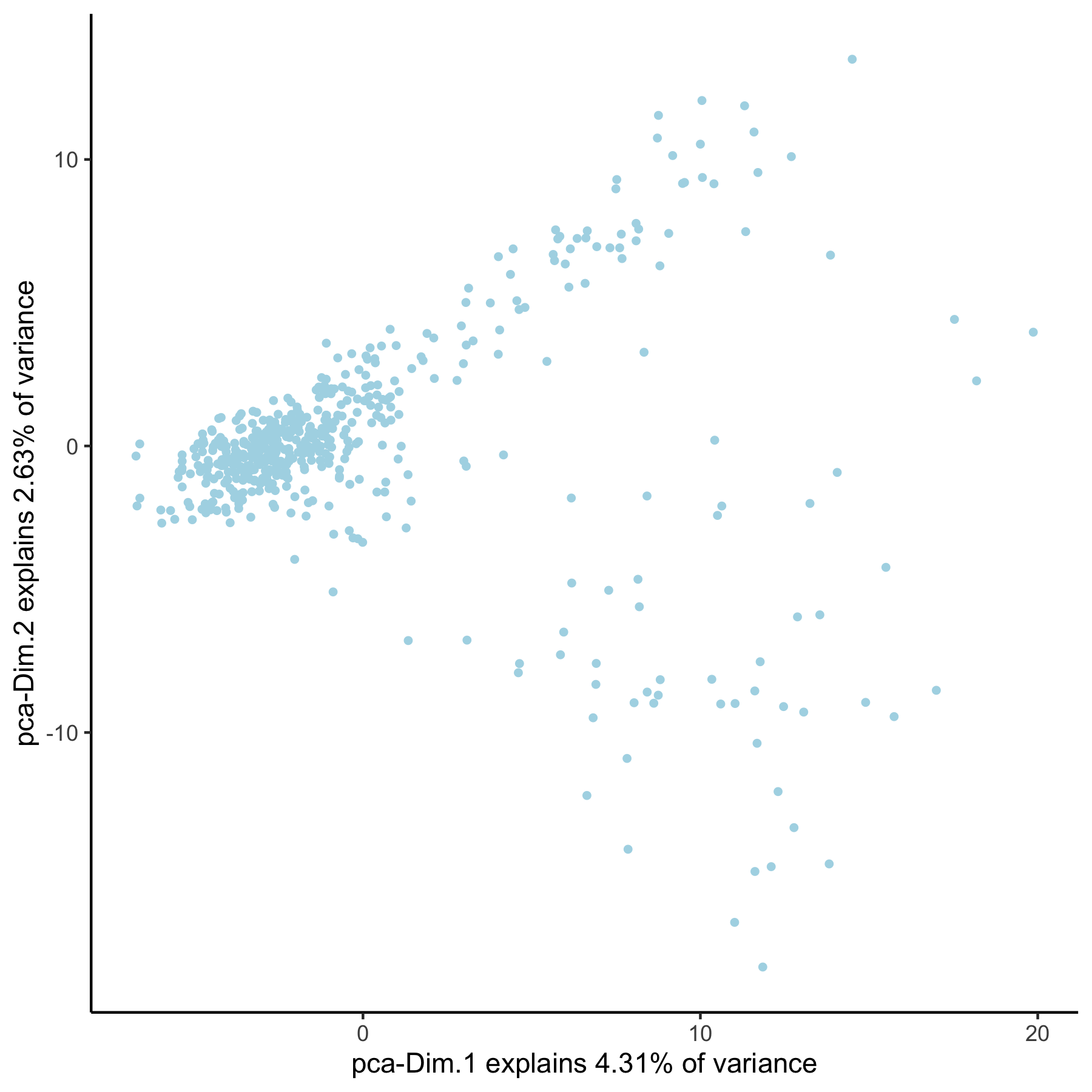```# pca with genes from group
VC_test <- runPCA(gobject = VC_test, genes_to_use = group_genes, name = 'pca_group', scale_unit = F)
plotPCA(gobject = VC_test, dim_reduction_name = 'pca_group')
```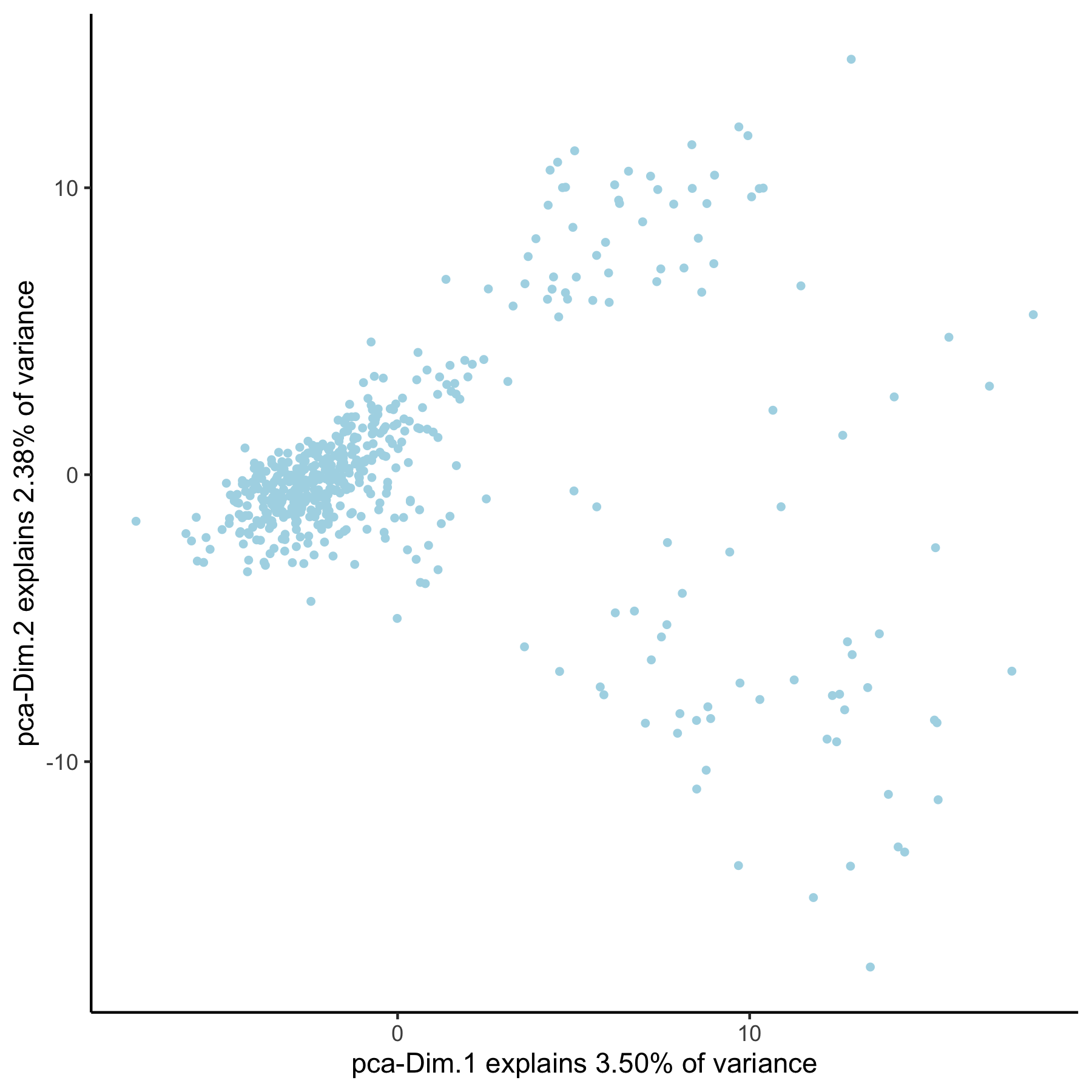## 3. Create Multiple UMAPs¶

• Using the 2 different PCA results (‘pca_loess’ and ‘pca_group’)

• Store UMAP results using custom names (‘umap_loess’ and ‘umap_group’)

• Plot UMAP results

```## 3. UMAP ##
VC_test <- runUMAP(VC_test, dim_reduction_to_use = 'pca', dim_reduction_name = 'pca_loess',
name = 'umap_loess', dimensions_to_use = 1:30)
plotUMAP(gobject = VC_test, dim_reduction_name = 'umap_loess')
```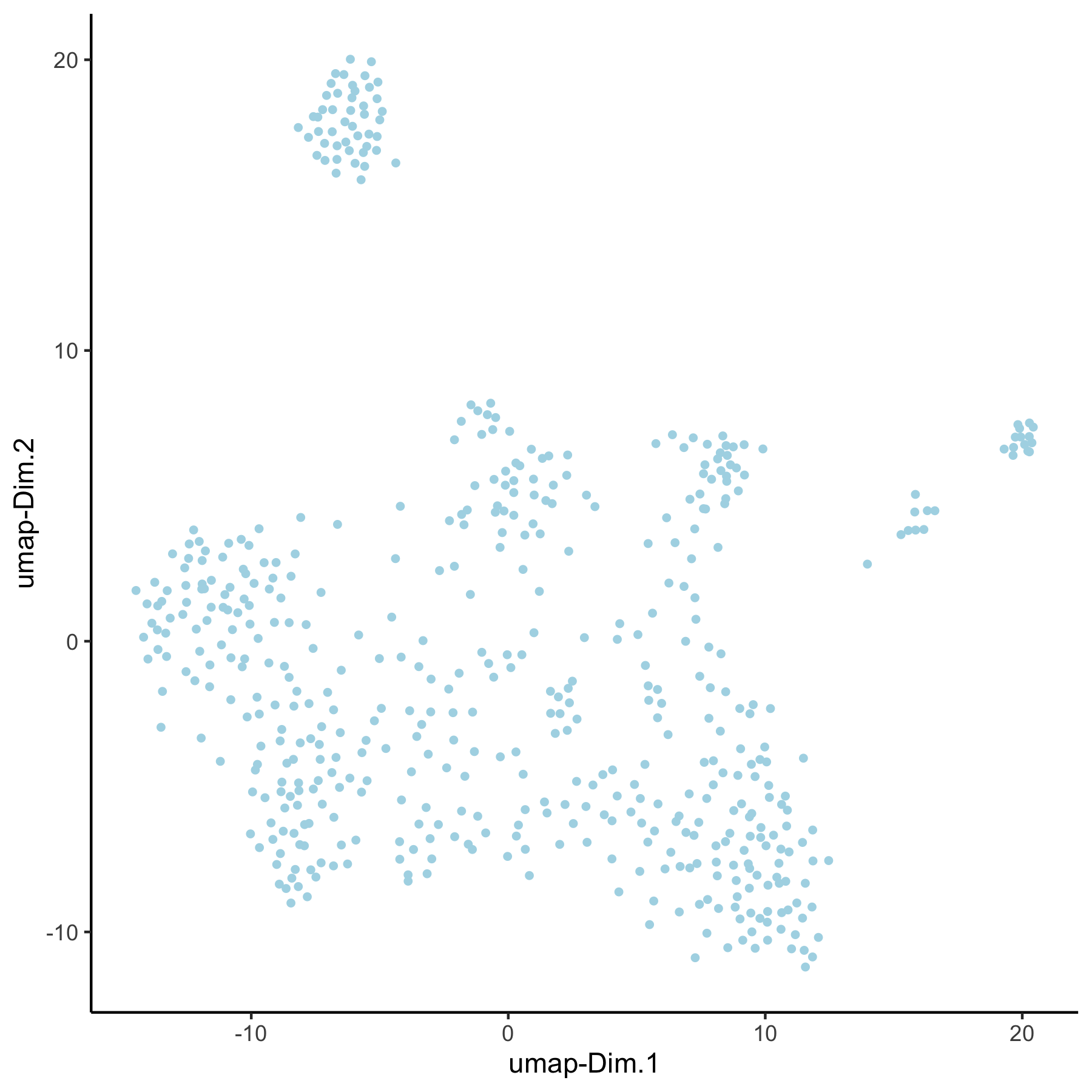```VC_test <- runUMAP(VC_test, dim_reduction_to_use = 'pca', dim_reduction_name = 'pca_group',
name = 'umap_group', dimensions_to_use = 1:30)
plotUMAP(gobject = VC_test, dim_reduction_name = 'umap_group')
```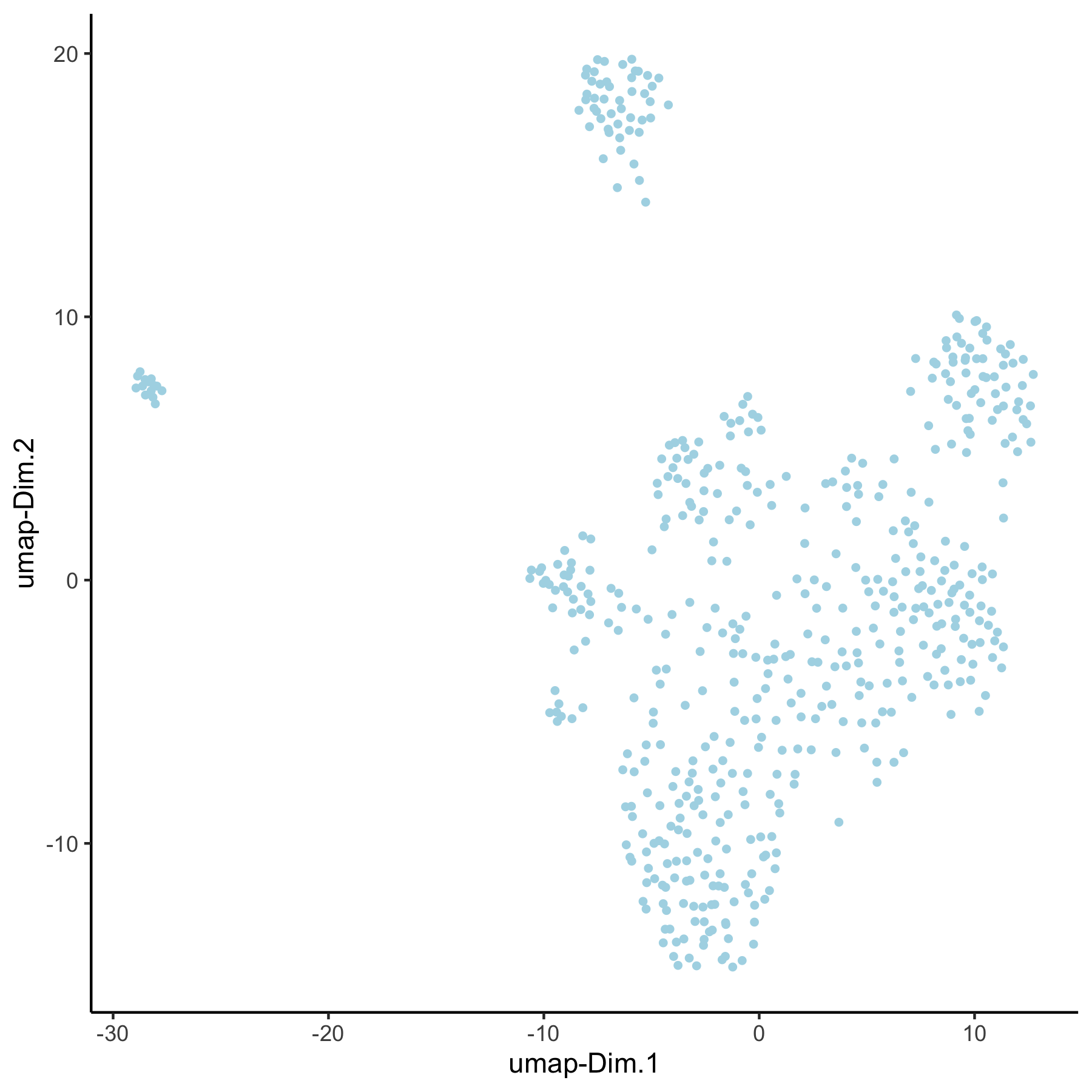## 4. Create Multiple Spatial Networks¶

• Create spatial with multiple k’s and other parameters (k=5, k=10, k=100 & maximum_distance=200)

• Subset field 1

• Visualize network on field 1 (‘spatial_network’, ‘large_network’, ‘distance_work’)

### Spatial Network¶

```## 4. spatial network
VC_test <- createSpatialNetwork(gobject = VC_test, method = 'kNN', k = 5) # standard name: 'spatial_network'
VC_test <- createSpatialNetwork(gobject = VC_test, method = 'kNN', k = 10, name = 'large_network')  VC_test <- createSpatialNetwork(gobject = VC_test, method = 'kNN', k = 100, maximum_distance_knn = 200, minimum_k = 2, name = 'distance_network')

## visualize different spatial networks on first field (~ layer 1)
subVC_test = subsetGiotto(VC_test, cell_ids = field1_ids)

spatPlot(gobject = subVC_test, show_network = T,
network_color = 'blue', spatial_network_name = 'spatial_network')
```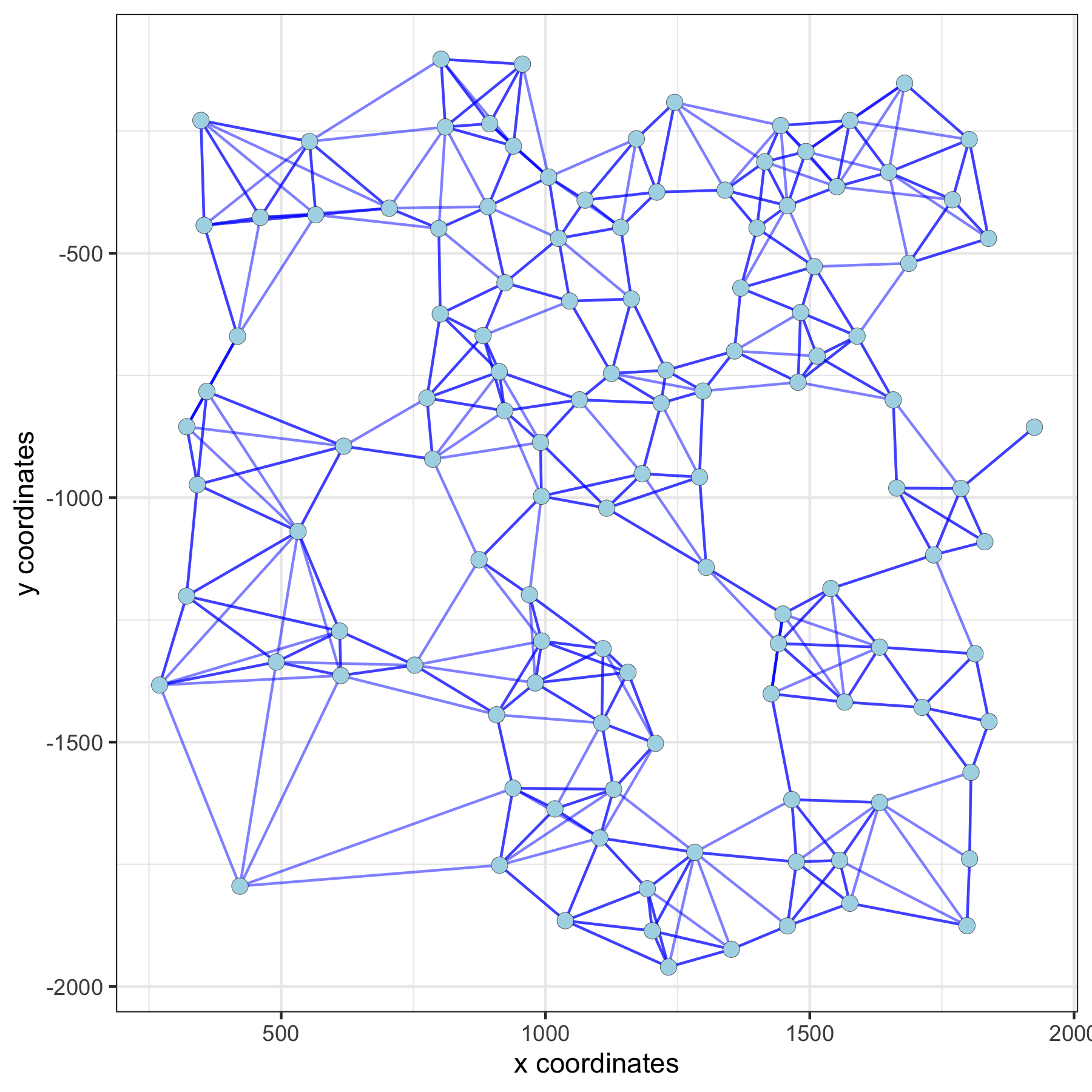### Large Network¶

```spatPlot(gobject = subVC_test, show_network = T,
network_color = 'blue', spatial_network_name = 'large_network')
```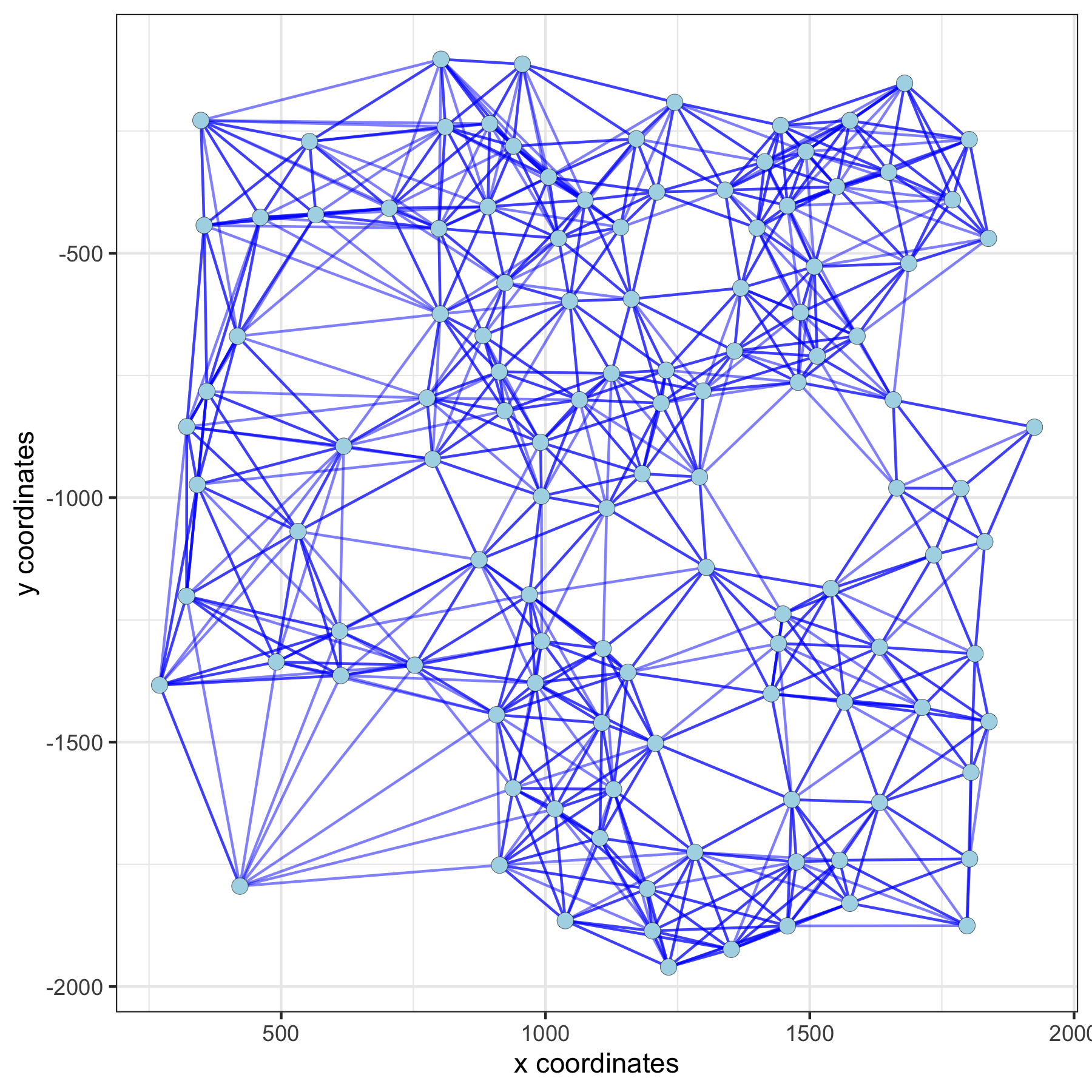### Distance Network¶

```spatPlot(gobject = subVC_test, show_network = T,
network_color = 'blue', spatial_network_name = 'distance_network')
```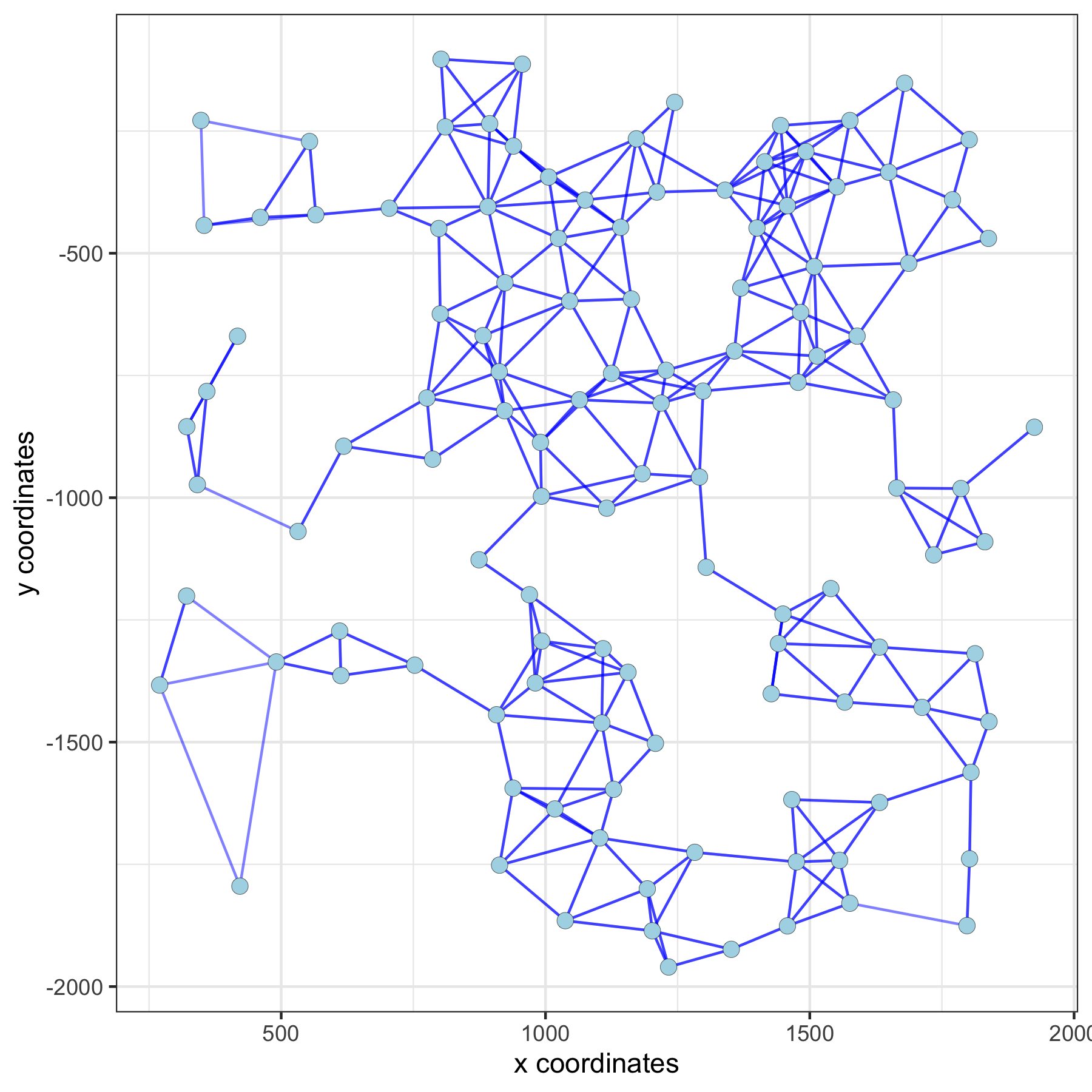## 5. Find Spatial Genes (Multiple Methods)¶

• Use the different spatial networks as input to identify spatial genes with the rank method

• Visualize top spatial genes for 2 methods

### Large Network Spatial Genes¶

```## 5. spatial genes
# the provided spatial_network_name can be given to downstream analyses

# spatial genes based on large network
ranktest_large = binSpect(VC_test,
subset_genes = loess_genes,
bin_method = 'rank',
spatial_network_name = 'large_network')

spatGenePlot(VC_test,
expression_values = 'scaled',
genes = ranktest_large\$genes[1:6], cow_n_col = 2, point_size = 1,
genes_high_color = 'red', genes_mid_color = 'white', genes_low_color = 'darkblue', midpoint = 0)
```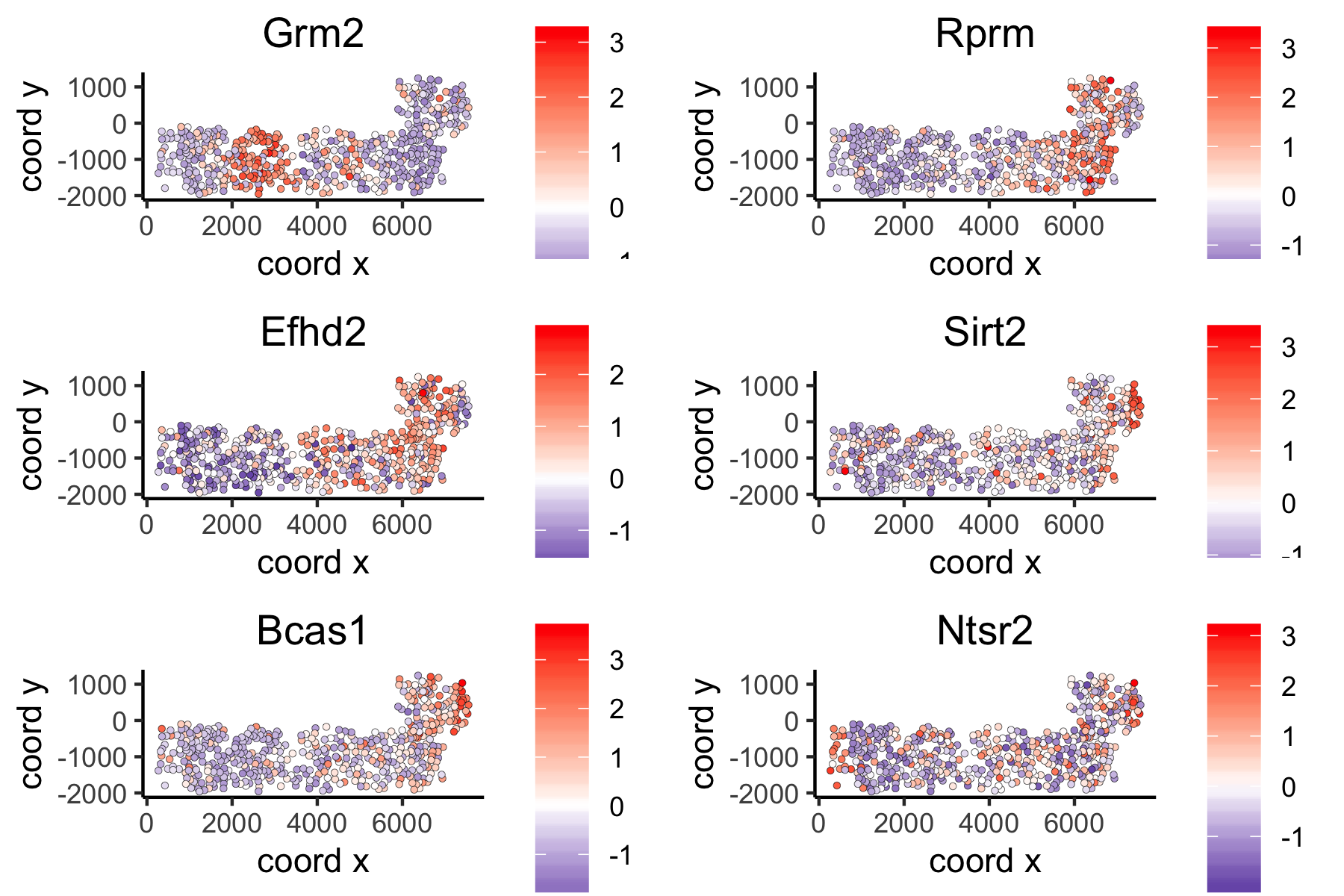### Distance Network Spatial Genes¶

```# spatial genes based on distance network
ranktest_dist = binSpect(VC_test,
subset_genes = loess_genes,
bin_method = 'rank',
spatial_network_name = 'distance_network')
spatGenePlot(VC_test,
expression_values = 'scaled',
genes = ranktest_dist\$genes[1:6], cow_n_col = 2, point_size = 1,
genes_high_color = 'red', genes_mid_color = 'white', genes_low_color = 'darkblue', midpoint = 0)
```